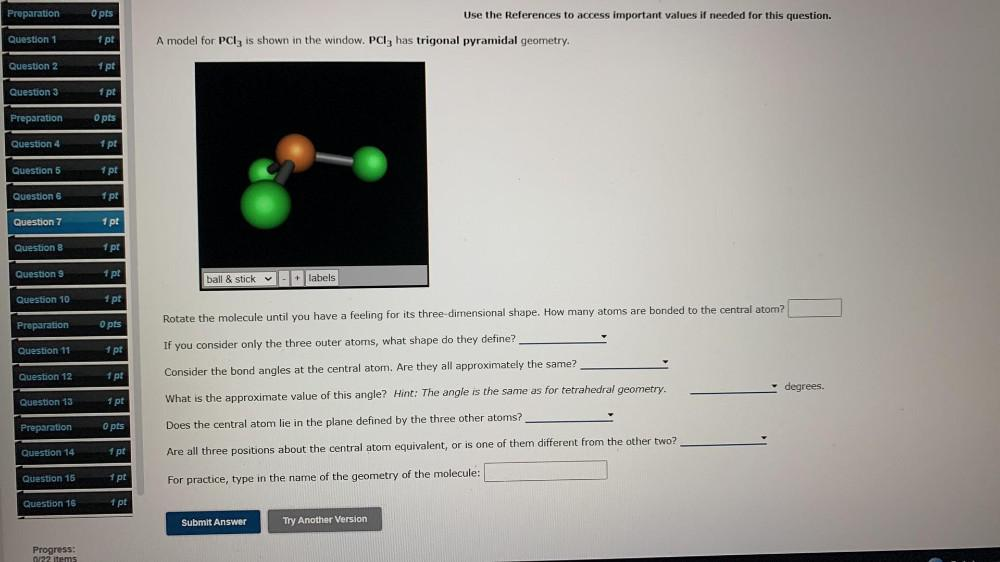Question:

# Preparation O pts Use the References to access important values if needed for this question. Question 1 1 pt A model for PC13 isPreparation O pts Use the References to access important values if needed for this question. Question 1 1 pt A model for PC13 is shown in the window. PC13 has trigonal pyramidal geometry. Question 2 1 pt Question 3 1 pt Preparation O pts Question 4 1 pt Question 5 1 pt Question 6 1 pt Question 7 1 pt Question 8 1 pt Question 9 1 pt ball & stick - + labels Question 10 1 pt opts Preparation Rotate the molecule until you have a feeling for its three-dimensional shape. How many atoms are bonded to the central atom? Question 11 1 pt If you consider only the three outer atoms, what shape do they define? Question 12 1 pt Consider the bond angles at the central atom. Are they all approximately the same? degrees. Question 13 1 pt What is the approximate value of this angle? Hint: The angle is the same as for tetrahedral geometry. Preparation O pts Does the central atom lie in the plane defined by the three other atoms? Question 14 1 pt Are all three positions about the central atom equivalent, or is one of them different from the other two? Question 15 1 pt For practice, type in the name of the geometry of the molecule: Question 16 1 pt Submit Answer Try Another Version Progress: 0/22 items